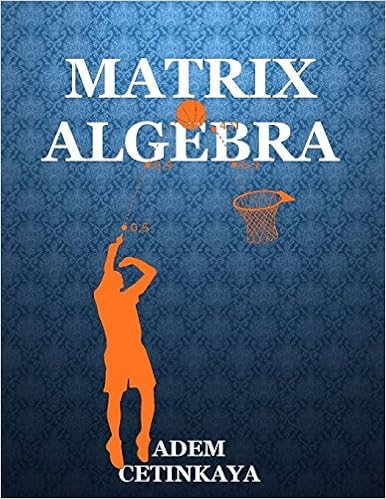Applications of matrices are found in most scientific fields. In every branch of physics, including classical mechanics, optics, electromagnetism, quantum mechanics, and quantum electrodynamics, they are used to study physical phenomena, such as the motion of rigid bodies. In computer graphics, they are used to manipulate 3D models and project them onto a 2-dimensional screen. In probability theory and statistics, stochastic matrices are used to describe sets of probabilities; for instance, they are used within the PageRank algorithm that ranks the pages in a Google search. Matrix calculus generalizes classical analytical notions such as derivatives and exponentials to higher dimensions. Matrices are used in economics to describe systems of economic relationships. A major branch of numerical analysis is devoted to the development of efficient algorithms for matrix computations, a subject that is centuries old and is today an expanding area of research. Matrix decomposition methods simplify computations, both theoretically and practically. Algorithms that are tailored to particular matrix structures, such as sparse matrices and near-diagonal matrices, expedite computations in finite element method and other computations. Infinite matrices occur in planetary theory and in atomic theory. A simple example of an infinite matrix is the matrix representing the derivative operator, which acts on the Taylor series of a function.We work across the world

From London to San Francisco, to our home base in (Saint Helier) Jersey, we’re looking for extraordinary and creative scientists to help us drive the field forward.

AC Investment Inc. currently does not act as an equities executing broker or route orders containing equities securities. If AC Invest’s business model were to change and it begins routing non-directed orders in NMS securities, it will comply with the disclosure requirement of Rule 606.

77 Massachusetts Avenue Cambridge, MA 02139 617-253-1000 pr@ademcetinkaya.com